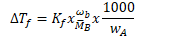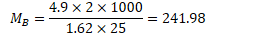# 2 g of benzoic acid C6H5COOH dissolved in 25 g of benzene shows a depression in freezing point equal to 1.62 K. Molal depression constant for benzene is 4.9 K kg mol−1. What is the percentage association of acid if it forms dimer in solution?

WB​=2g,

Kf​=4.9K Kgmol−1

WA​=25g,

ΔTf​=1.62K

nowIf x is the degree of association, (1−x) mole of benzoic acid left undissociated & corresponding x/2 as associated moles of C6​H5​COOH at equilibrium.

i = Normal molecular mass / Abnormal molecular mass

1-x/2 = 122/241.98

x=0.992 = 99.2%